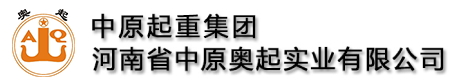全国统一服务热线:13782575673

# 龙门吊起重机大车的主梁设计计算

### 来源：本站   发布时间：2021-03-15 02:42:52  点击量：38

①设计计算主梁梁高

运行冲击系数取φ4=1.16;起升冲击系数取φ2=1.14;额定起重量Q=320000N;小车自重重量GXC=40000N(小车自重重量参照同类小车的自重，对没有参照的小车自重，可先估算，待设计完小车后，再回过头来验算主梁的设计计算);小车基距b=980mm;主梁跨度S=22500mm;

截面腹板厚度之和：δ=16mm，δ1=8mm，δ2=8mm采用Q235材料;

许用应力：[σ]=1.40×105kN/m2;弹性模量：E=2.1×108kN/m2;

许用刚度：推荐初选设计主梁：β=700(A5级以下);

腹板加强劲重量比腹板重量：α=1/3;

走台栏杆轨道机械电气设备重量比主梁重量：λ=0.2;

材料比重：γ=78.5kN/m3;

C1为将小车轮压转化为跨中集中力时计算弯矩的换算系数，C1=1;

C2为将小车轮压转化为跨中载荷计算挠度的换算系数：[Q]>Q=320kN，刚度为控制条件。

经济梁高：圆整取：hg=1150mm(作为主梁上下盖板之间的净高)

②初步确定主梁截面面积

经济梁高上盖板与腹板截面积之比取：?上盖板的截面积尺寸：?上盖板的厚度：δ3=12mm

下盖板的厚度：δ4=12mm

上盖板的宽度：?圆整取：by=500mm，作为主梁腹板之间的净宽。

③确定承压梁的梁高

根据前面计算和用户给定参数得：φ2=1.14;φ4=1.16;GXC=40000N;Gq=170000N;Q=320000N;δ1=δ2=8mm;δ3=12mm;δ4=20mm;B2=576mm;[τⅠ]=140/1.732=80MPa;Ix=2.3×109mm4。

考虑制作的工艺性，承压梁梁高按经验公式取值。

h1=(0.25～0.5)h;B1=(1.2～1.6)B2;取h1=300mm，B1=876mm

则Sx=4059680mm3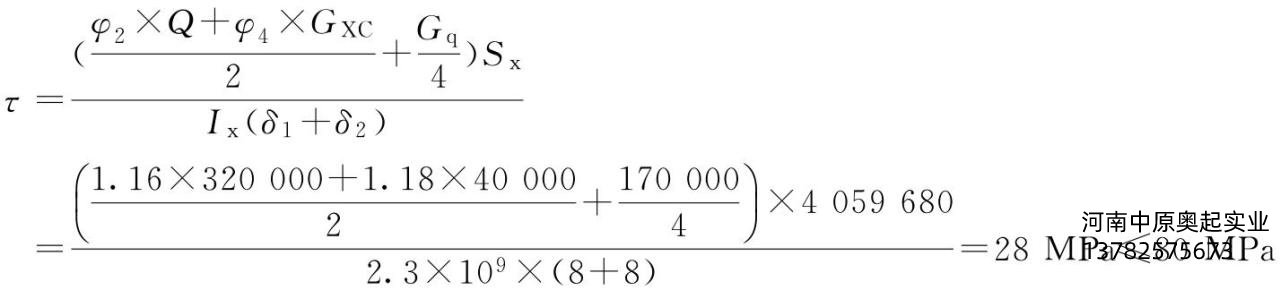所选承压梁梁高和截面符合要求。

④主梁的筋板布置

主梁采取半偏轨梁，对腹板120<1150/8=144≤160，根据腹板的加劲原则，在梁全长内设置横向大加筋板和小三角筋板，并同时在受压区设置一道和第二道纵向加劲杆。第一道高度设置在距腹板受压边缘(0.2～0.3)h0处，第二道设置在距腹板受压边缘(0.5～0.7)h0处;主梁大隔板间距(除两端尺寸外)a=2000mm，省去小隔板，采用小三角筋板。h0=1150，取第一道高度为300mm，第二道高度在距腹板受压边缘800mm。

对上盖板，508/12=42≤50，根据盖板的加劲原则，上盖板不需布置纵向加劲杆。

⑤对主梁进行Ⅲ类载荷组合验算

两腹板中心线间距：b1=508mm;

主梁高度：h0=1150mm(净空);

大车加减速：a=0.15m/s2;

半个大车的结构重量：Gq=84000N(主梁+走台);

主梁走台自重均布载荷：?均布载荷在跨中引起的最大弯矩：?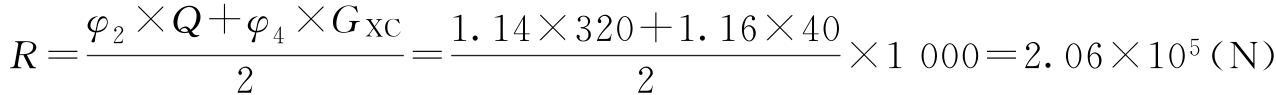作用于一根主梁上的载荷：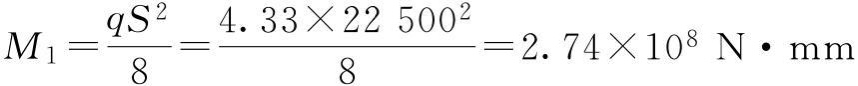在设计小车时，得a1=490mm，a2=490mm;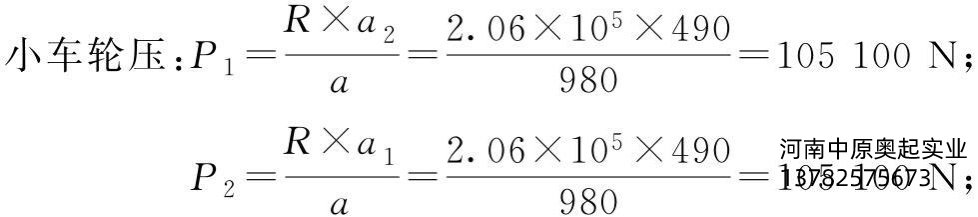主梁截面几何参数：Ix=7.52×109mm4;y1=629mm;y2=545mm;Iy=1.62×109mm4;x1=299mm;x2=277mm。

a.主梁垂直静挠度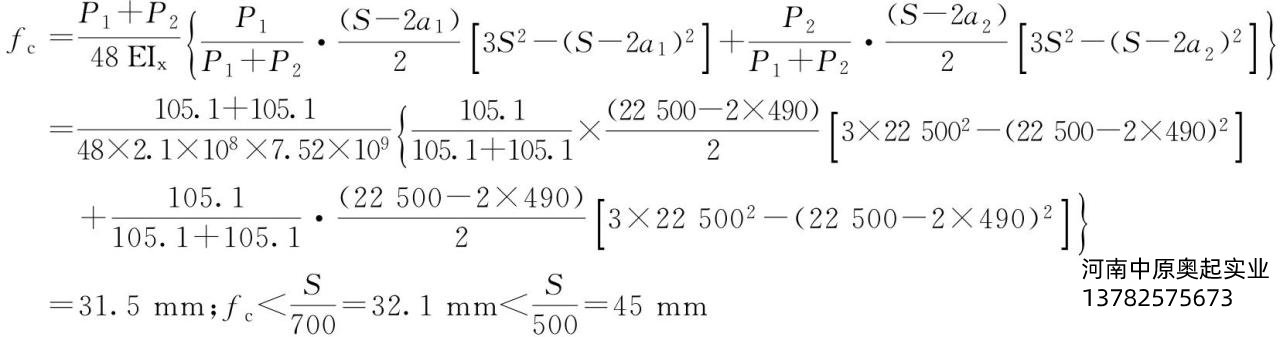垂直刚度满足设计要求。

b.垂直平面内的应力计算

?集中力引起的跨中最大弯矩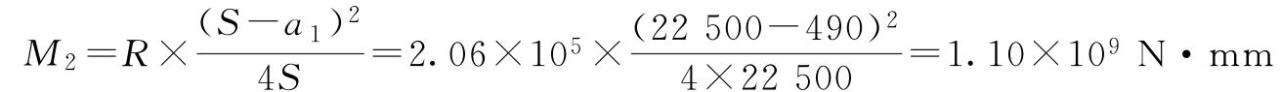垂直平面内主梁跨中截面正应力

c.水平平面内的应力计算(主梁惯性载荷产生的弯矩)

?均布惯性载荷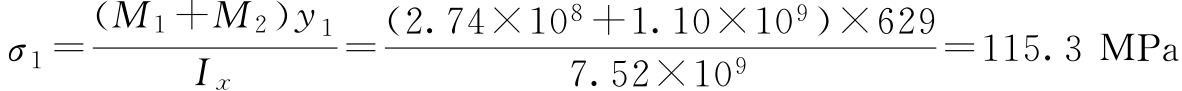均布惯性载荷跨中产生的最大弯矩：?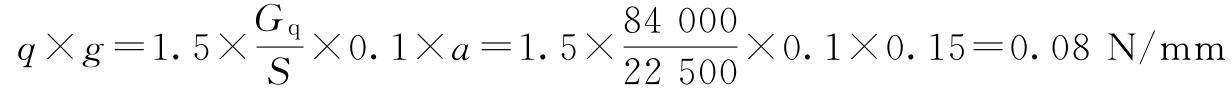小车对主梁的集中惯性载荷

Pg=1.5(GXC+Q)×0.1×0.5×a=1.5×(4+32)×104×0.1×0.5×0.15=4.05×103(N)

集中惯性载荷引起的跨中最大弯矩：水平平面内主梁跨中截面正应力：

综合应力：σ=1.15(σ1+σ2)=1.15(115.3+5.00)=138.34MPa≤[σ]=140MPa式中，1.15为考虑主梁跨中的约束扭转和约束弯曲的影响，强度满足设计要求。水平刚度满足设计要求。